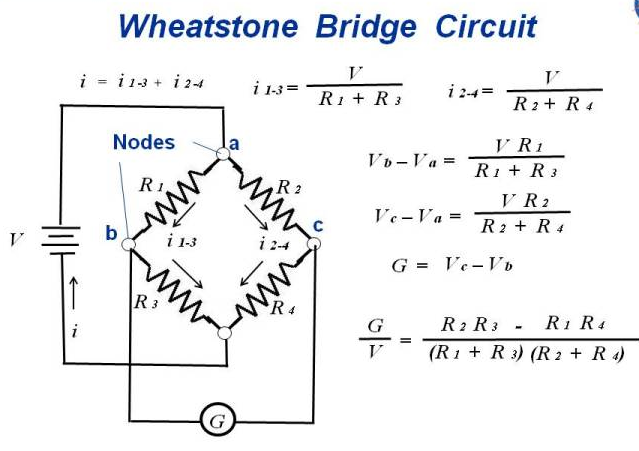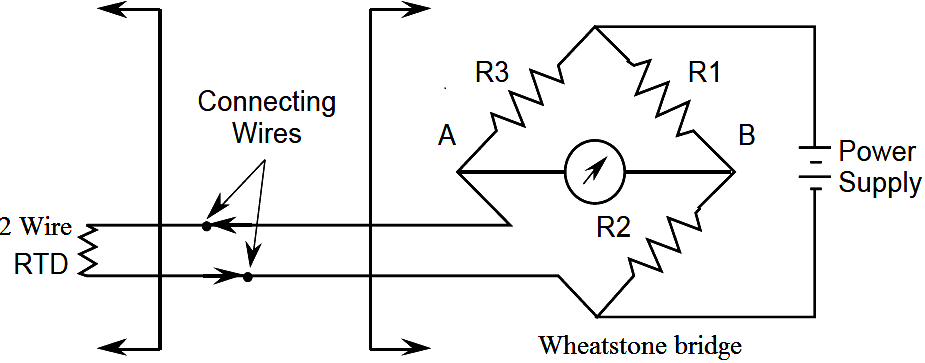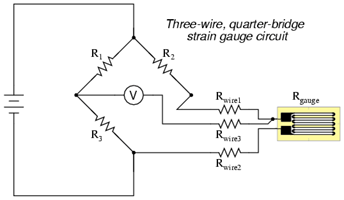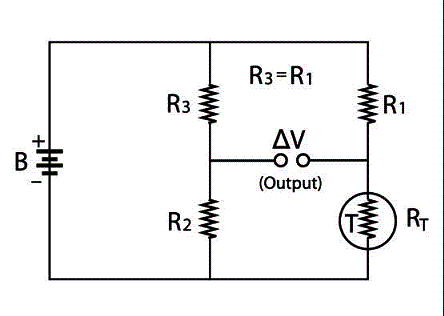Wheatstone Bridge for JEE Physics

Principle, Construction, And Working Of The Wheatstone Bridge for IIT JEE Exam

Wheatstone bridge is also referred to as the resistor bridge. It is used for the calculation of unknown resistance by balancing two legs of abridge circuit where one of the two legs consists of a component having an unknown resistance.

It is used as a means of calibrating the measuring instruments such as voltmeters, ammeters, etc. Though the digital multi-meters today are the simplest way to measure resistance, the Wheatstone bridge is still used to measure the resistance of very low range in milli-ohms.

The Wheatstone bridge was first found by Samuel Hunter Christie in 1883 and then was made famous by Sir Charles Wheatstone in the year 1843.

PRINCIPLE, CONSTRUCTION, and WORKING OF THE WHEATSTONE BRIDGE
BRIDGE:

Wheatstone works on the principle of null detection i.e. the ratio of the resistors are equal and no current flows through it.
Under normal conditions, the bridge is said to be unbalanced where the current flows through the galvanometer. When the bridge is balanced, no current flows through the galvanometer. This condition is achieved by balancing the known resistance and the variable resistance.

The basic circuit of the Wheatstone bridge is as shown in the figure below.
The bridge has four arms which consist of two known resistance, one variable resistanceR4, and one unknown resistance R3.In the figure above we see that the resistors are connected to each other at nodes a, b and c. The potentiometer labeled G is used to detect the voltage difference between nodes c and b.

If we consider R1, R2, R3 and R4, each contain its own current (i1, i2, i3 and i4) and voltage (V1, V2, V3, V4) respectively are related to each other by ohm’s law.

Resistor R1 and R3 are connected in series through node b so the same current flows through R1and R3.
i( 1-3) = i1=i3 = V/ (R1+R3)…………….. 1

Similarly,
i( 2-4)= i2=i4 = V/(R2+R4)………………….. 2

The equal current flows through R1 and R2. The following conditions occur when the current through the galvanometer is zero.

i1R1= i2R2………..3

Substituting 1 and 2 in the equation 3, we get

V/ (R1+R3)*R1 = V/ (R2+R4)*R2

R1/ (R1+R3) = R2/ (R2+R4)
R1(R2+R4) = R2(R1+R3)
R1R2 + R1R4 = R1R2 + R3R2
R1R4 = R3R2……….4
R4 = R3R2/R1……….5

Equation 4 shows the balanced condition of the bridge while equation 5 determines the value of the variable.
In the above diagram, R4 is the unknown arm.

LIMITATION OF WHEATSTONE BRIDGE:

• • The Wheatstone bridge also has some limitations as follows:

• • The Wheatstone bridge fails if it is not in balanced condition.

• • It is able to produce results from few ohms to few mega-ohms.

• • Some personal errors may also occur while handling the Wheatstone bridge.

• • The Wheatstone bridge may also get affected if the galvanometer is not of a good quality

• • The error may occur if the three of the resistor reading is not recorded properly.

• APPLICATION OF THE WHEATSTONE BRIDGE

The Wheatstone bridge is used to measure the physical quantities such as stress, strain, temperature, etc., with the help of an operational amplifier.

RTD IN WHEATSTONE BRIDGE

The resistance temperature detectors are used to detect the changes in the temperature of the metal wires. We all know that every metal has its own resistivity constant. That is every metal has its own resistance power to resist the flow of electrons.

This resistance power is measured in the form of the changes in temperature with the help of resistor temperature detectors.
This change in resistance is sometimes in linearly proportional to the change in temperature. This coefficient is called a
Temperature coefficient of electrical resistance (TCR). Many of the metal wires are used as the RTD for the measurement of resistance. Platinum is used widely as the sensor because of its linearity, whereas gold and silver are least used because they are good conductors of electricity.As shown in the above figure the Wheatstone bridge is connected to the RTD. It is the two-wire RTD connected to the
Wheatstone bridge through the transmitting wires. The three resistors in the Wheatstone bridge are already known. In the case of the thermocouple, the RTD is placed away from the transmitter with the help of the connecting wires.

The limitation in using RTD is the RTD needs to be separated from the Wheatstone bridge with the help of the connecting wires so as to avoid the non-linear relationship between resistance change and the voltage output change.

STRAIN GAUGE IN WHEATSTONE BRIDGE

When the strain gauge is installed in the Wheatstone bridge then the Wheatstone bridge converts the applied force, pressure and strain in the electrical resistance. Hence, the applied force can be measured in the form of resistance with the help of Wheatstone bridge. We know that when the wire is elongated due to strain or elongation, the diameter of the wire decreases.
So when the diameter increases, the length increases and the resistance decreases. Hence, the principle of working of the strain gauge is that the resistance of the conductor is inversely proportional to the length of the conductor that is when the length increases, the resistance decreases.The diagram above shows the strain gauge used with the Wheatstone bridge. The voltmeter will show the null deflection till there is no strain or force applied to the strain gauge. As the force on the strain gauge is increased, the resistance is decreased.
Strain gauges also have some limitations. So the strain gauges must be checked thoroughly in terms of fatigue environment and previous strain before using for the measurement.

TERMISTOR IN WHEATSTONE BRIDGE

This is also one of the important applications of the Wheatstone bridge to detect the change in temperature. A Thermistor is one of the best things used in temperature related applications. The word itself indicates ‘therm’ that is thermal which means related to temperature and ‘istor’ that is a resistor which means related to resistance. Therefore, the thermistors are used for the precise and accurate results for the applications. Hence, by using the negative temperature coefficient graph technique one can easily find out the precise temperature by putting the thermistor in one leg of the Wheatstone bridge. If we need to compare the two readings then we can put two thermistors in two legs of the Wheatstone bridge. Hence, thermistors are used in the differential thermometers and can be placed in various points and compared with the original one. The thermistor can be used to control the temperature using the temperature resistance curve using the AC bridge.The above figure shows the Wheatstone bridge using the thermistor to detect the temperature difference. When the temperature will be out of balance, the voltage will be detected by V. Then by using temperature resistance graph diagram, we can measure the accurate temperature.

• • The main advantage of the Wheatstone bridge is that it can be easily interfaced into various combinations.

• • The Wheatstone bridge is traditionally called ohmmeter as the results are measured in terms of resistance and also are accurate and precise.

• • We can measure minute changes in the bridge, even in m ohms’.

• • It is very easy to find out the unknown resistance as the rest of the three are easily known.

• • The disadvantage of the Wheatstone bridge is that it is not so precise when it is used to measure large resistances.

• • When the large resistances are to be measured, the results are mostly non-linear.

• • Sometimes the value of the resistance degrades depending on the temperature and the EMF applied.

• The combination of four resistance connected to a null center is called the Wheatstone Circuit Bridge. The Wheatstone Bridge gets its name from Charles Wheatstone who developed this bridge to measure the unknowns resistance values and for calibrating the measuring instruments. However, the invention of multimeters has made it very easy to measure the resistance without many efforts. However, there are some areas where still the Wheatstone Circuit Bridge is used to measure the resistance. In order to interface several transducers and sensors, one can use the Wheatstone Bridge Circuit. The Wheatstone Circuit Bridge is used widely in electronic circuits. It is also used in Operational Amplifiers to amplify and measure any small changes in the resistance. The Circuit Bridge is also helpful to measure changes in resistance with respect to other changing quantities. It is very important to understand the implications and usage of the Wheatstone Circuit Bridge to be well acquainted with the concept of resistance. The bridge is used to find the value of an unknown resistance with the known resistors, Galvanometer etc. Since the last 20 years, the Wheatstone Circuit bridge is being used for test applications, sensors, topology etc.